# RZ.eqvolt-asors8.46.n for Problem 8.52. For three circuit element os for binations shown in the figure below,find Zeg given that wL = 50 mH, R = 1 kQ.com-2000 rad/s,C = 5 µF,uita)eqCL,b)ACRsoreqom-c)Probleof anyL.C.C.C.

Question
1 views

For the three circuit elelents in the image find Zeq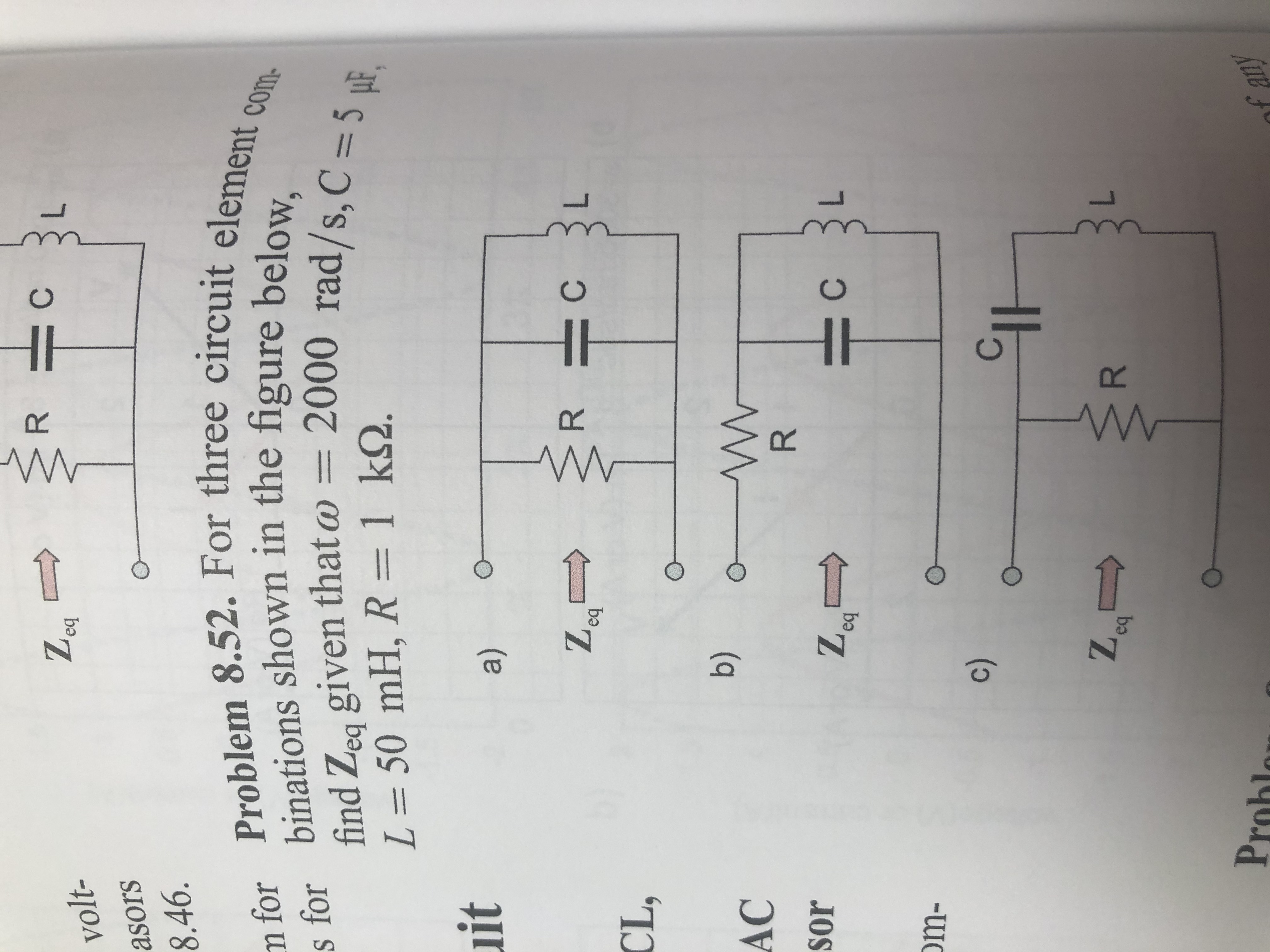help_outlineImage TranscriptioncloseR Z. eq volt- asors 8.46. n for Problem 8.52. For three circuit element o s for binations shown in the figure below, find Zeg given that w L = 50 mH, R = 1 kQ. com- 2000 rad/s,C = 5 µF, uit a) eq CL, b) AC R sor eq om- c) Proble of any L. C. C. C. fullscreen
check_circle

Step 1

The impedance offered by the inductor and the capacitor is given by,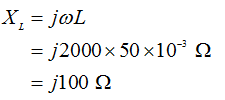And,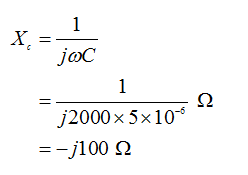Step 2

Part (a)

All the elements are connected in parallel. So, the equivalent resistance can be expressed by the following expression.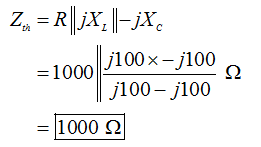NOTE:- Here, the parallel combination of L and C will offer infinite impedance, so the current will only flow through the resistor. Hence, the Thevenin impedance will be equal to the value of R.

Step 3

Part (b)

For this configuration, the resistor is in series with the parallel combination of L and C.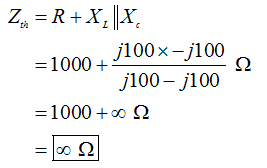The given circuit will behave as an open circuit.

...

### Want to see the full answer?

See Solution

#### Want to see this answer and more?

Solutions are written by subject experts who are available 24/7. Questions are typically answered within 1 hour.*

See Solution
*Response times may vary by subject and question.
Tagged in